# Grouped, stacked and percent stacked barplot in ggplot2

This post explains how to build grouped, stacked and percent stacked barplots with R and ggplot2. It provides a reproducible example with code for each type. Note that this online course has a dedicated section on barplots using the `geom_bar()` function.

# Grouped barchart

A grouped barplot display a numeric value for a set of entities split in groups and subgroups. Before trying to build one, check how to make a basic barplot with `R` and `ggplot2`.

A few explanation about the code below:

• input dataset must provide 3 columns: the numeric value (`value`), and 2 categorical variables for the group (`specie`) and the subgroup (`condition`) levels.
• in the `aes()` call, x is the group (`specie`), and the subgroup (`condition`) is given to the `fill` argument.
• in the `geom_bar()` call, `position="dodge"` must be specified to have the bars one beside each other.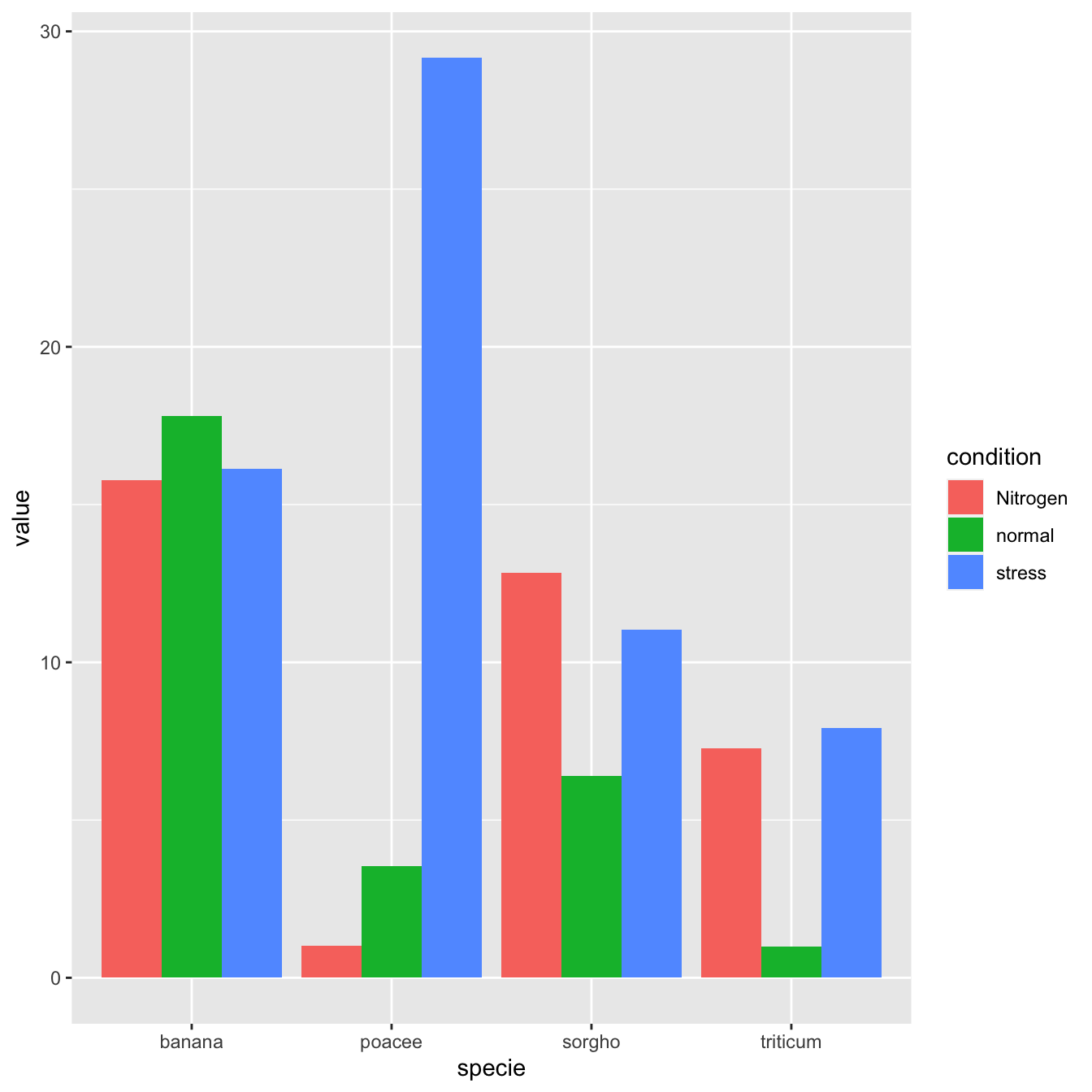`````` # library
library(ggplot2)

# create a dataset
specie <- c(rep("sorgho" , 3) , rep("poacee" , 3) , rep("banana" , 3) , rep("triticum" , 3) )
condition <- rep(c("normal" , "stress" , "Nitrogen") , 4)
value <- abs(rnorm(12 , 0 , 15))
data <- data.frame(specie,condition,value)

# Grouped
ggplot(data, aes(fill=condition, y=value, x=specie)) +
geom_bar(position="dodge", stat="identity")``````

# Stacked barchart

A stacked barplot is very similar to the grouped barplot above. The subgroups are just displayed on top of each other, not beside.

The only thing to change to get this figure is to switch the `position` argument to `stack`.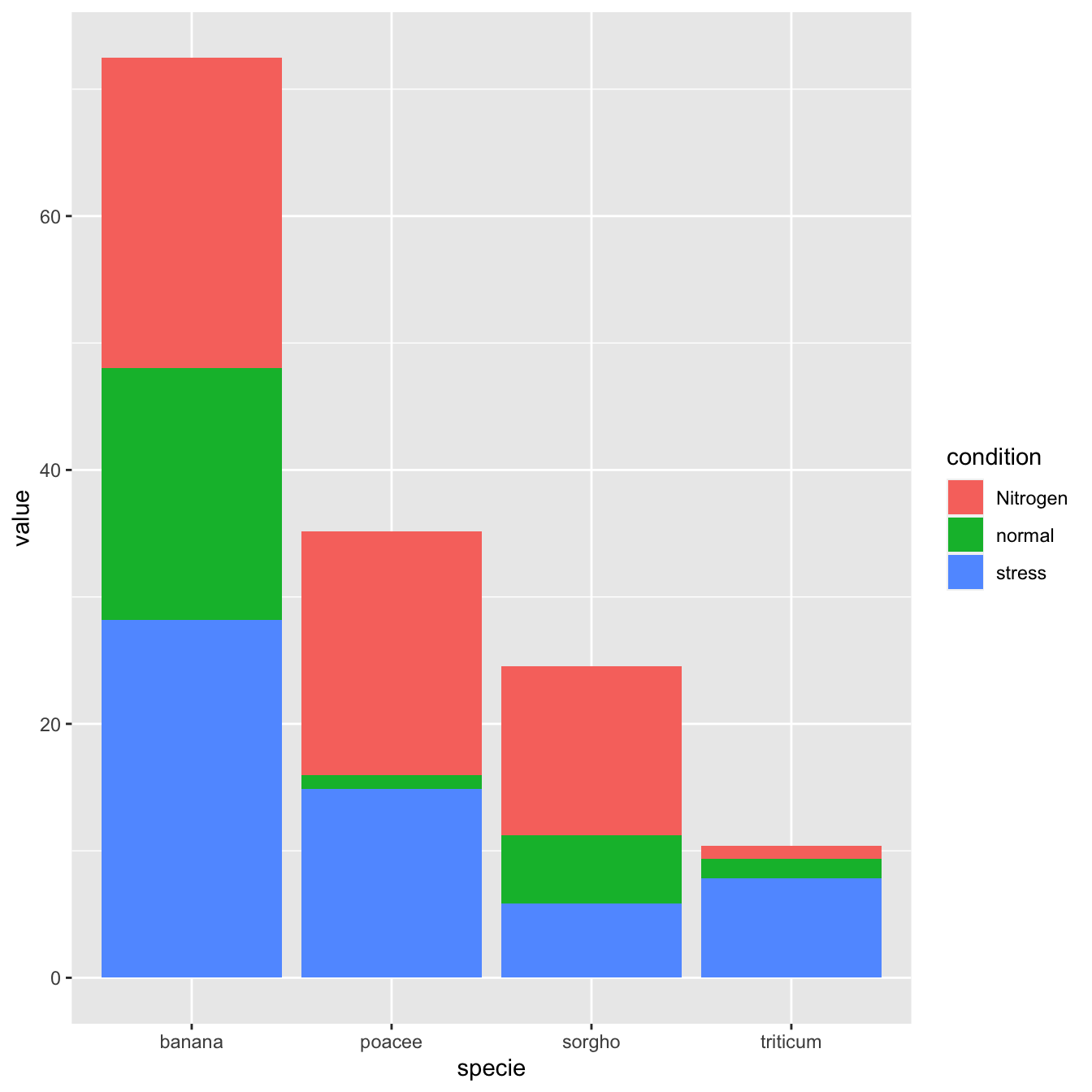`````` # library
library(ggplot2)

# create a dataset
specie <- c(rep("sorgho" , 3) , rep("poacee" , 3) , rep("banana" , 3) , rep("triticum" , 3) )
condition <- rep(c("normal" , "stress" , "Nitrogen") , 4)
value <- abs(rnorm(12 , 0 , 15))
data <- data.frame(specie,condition,value)

# Stacked
ggplot(data, aes(fill=condition, y=value, x=specie)) +
geom_bar(position="stack", stat="identity")``````

# Percent stacked barchart

Once more, there is not much to do to switch to a percent stacked barplot. Just switch to `position="fill"`. Now, the percentage of each subgroup is represented, allowing to study the evolution of their proportion in the whole.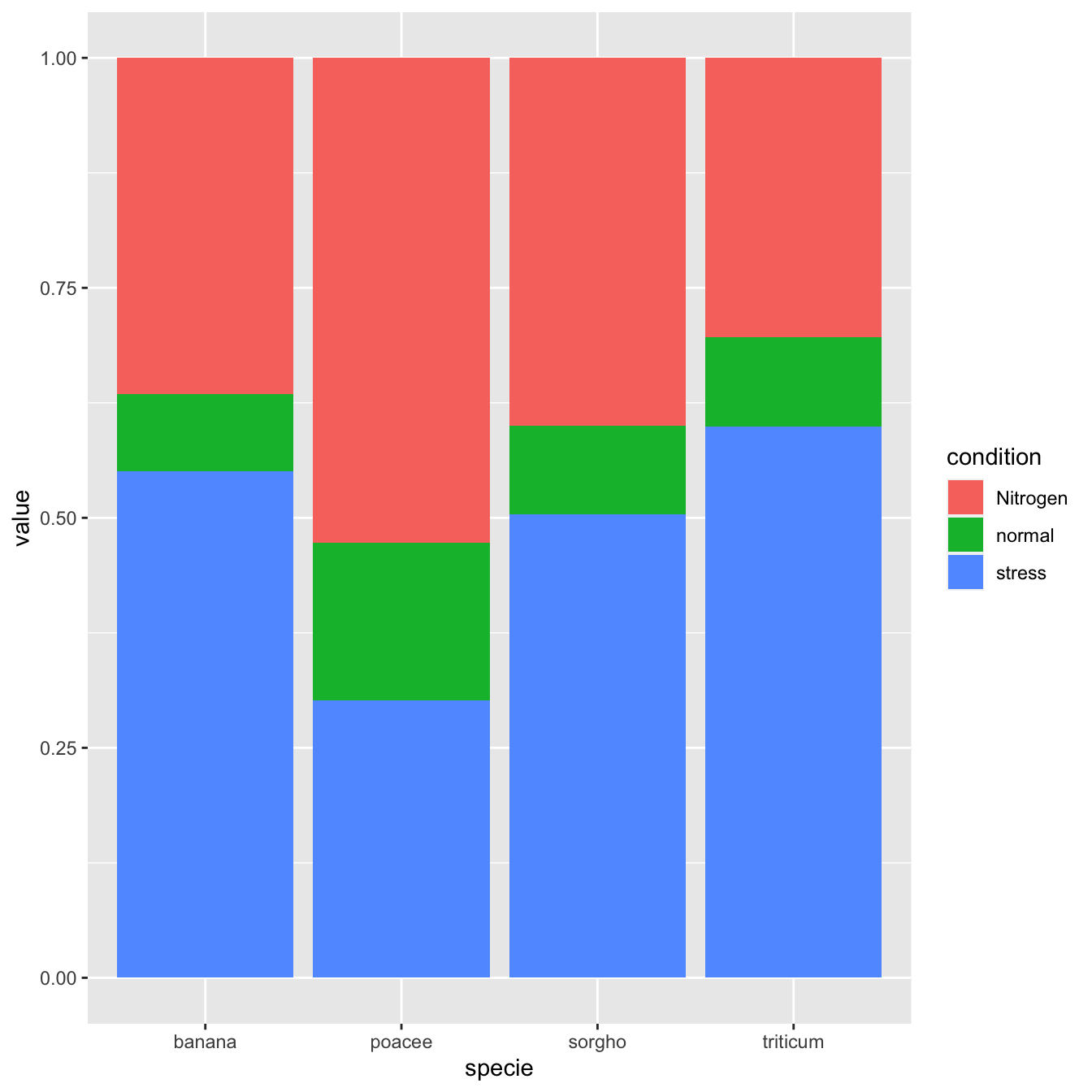`````` # library
library(ggplot2)

# create a dataset
specie <- c(rep("sorgho" , 3) , rep("poacee" , 3) , rep("banana" , 3) , rep("triticum" , 3) )
condition <- rep(c("normal" , "stress" , "Nitrogen") , 4)
value <- abs(rnorm(12 , 0 , 15))
data <- data.frame(specie,condition,value)

# Stacked + percent
ggplot(data, aes(fill=condition, y=value, x=specie)) +
geom_bar(position="fill", stat="identity")``````

# Grouped barchart customization

As usual, some customization are often necessary to make the chart look better and personnal. Let’s:

• use a theme
• change color palette. See more here.
• customize axis titles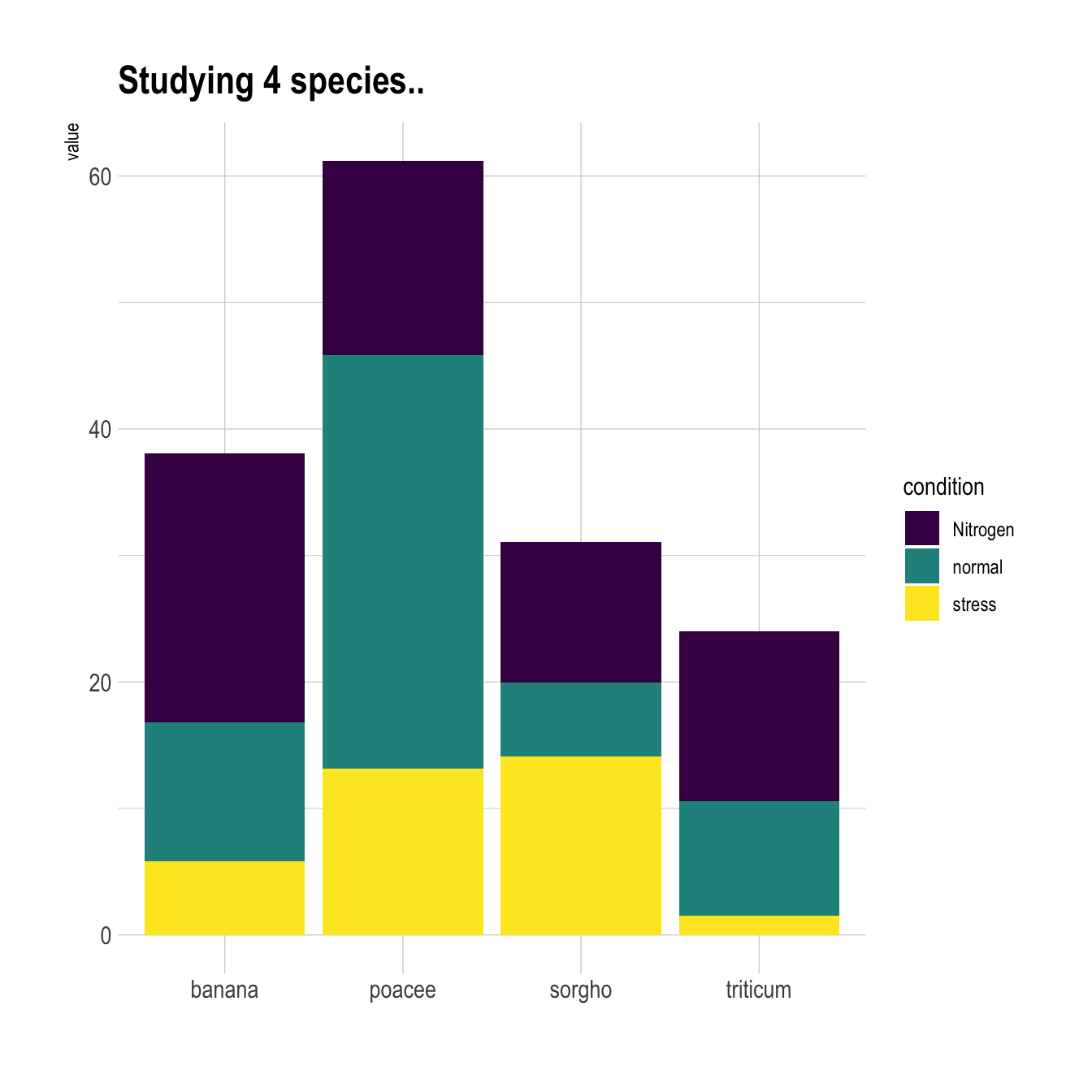``````# library
library(ggplot2)
library(viridis)
library(hrbrthemes)

# create a dataset
specie <- c(rep("sorgho" , 3) , rep("poacee" , 3) , rep("banana" , 3) , rep("triticum" , 3) )
condition <- rep(c("normal" , "stress" , "Nitrogen") , 4)
value <- abs(rnorm(12 , 0 , 15))
data <- data.frame(specie,condition,value)

# Small multiple
ggplot(data, aes(fill=condition, y=value, x=specie)) +
geom_bar(position="stack", stat="identity") +
scale_fill_viridis(discrete = T) +
ggtitle("Studying 4 species..") +
theme_ipsum() +
xlab("")``````

# Small multiple

Small multiple can be used as an alternative of stacking or grouping. It is straightforward to make thanks to the `facet_wrap()` function.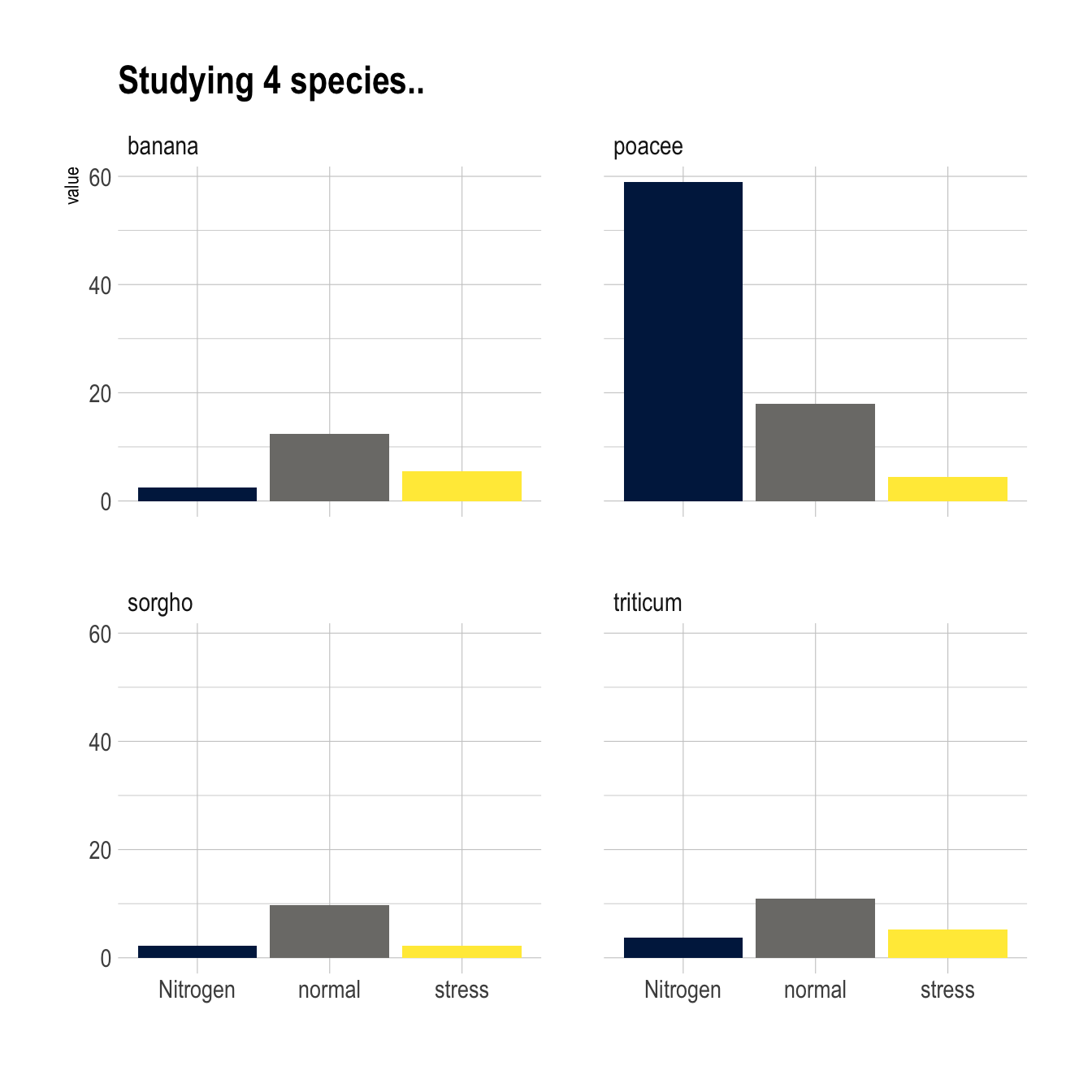``````# library
library(ggplot2)
library(viridis)
library(hrbrthemes)

# create a dataset
specie <- c(rep("sorgho" , 3) , rep("poacee" , 3) , rep("banana" , 3) , rep("triticum" , 3) )
condition <- rep(c("normal" , "stress" , "Nitrogen") , 4)
value <- abs(rnorm(12 , 0 , 15))
data <- data.frame(specie,condition,value)

# Graph
ggplot(data, aes(fill=condition, y=value, x=condition)) +
geom_bar(position="dodge", stat="identity") +
scale_fill_viridis(discrete = T, option = "E") +
ggtitle("Studying 4 species..") +
facet_wrap(~specie) +
theme_ipsum() +
theme(legend.position="none") +
xlab("")``````

Related chart types

## Contact

This document is a work by Yan Holtz. Any feedback is highly encouraged. You can fill an issue on Github, drop me a message on Twitter, or send an email pasting yan.holtz.data with gmail.com.